## A line passes through the points (6,-6) and (9,-5). What is it’s equation in point slope form?

Question

A line passes through the points (6,-6) and (9,-5). What is it’s equation in point slope form?

in progress 0
2 weeks 2021-09-02T08:39:20+00:00 1 Answers 0 views 0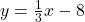Step-by-step explanation:

Slope-intercept form of an equation is written as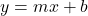, where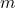is the slope and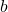is the y-intercept.

The slope of a line that passes through the points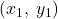and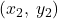is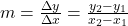. Using the coordinates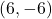and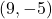as given in the problem, we have slope of this line to be: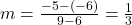.

Now using this slope we’ve found and any point the line passes through, we can find the y-intercept of this equation: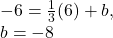Therefore, the equation of this line in slope-intercept form is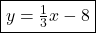.